# HTRU1

HTRU1 Batched Dataset

View the Project on GitHub as595/HTRU1

# HTRU1

The HTRU1 Batched Dataset is a subset of the HTRU Medlat Training Data, a collection of labeled pulsar candidates from the intermediate galactic latitude part of the HTRU survey. HTRU1 was originally assembled to train the SPINN pulsar classifier. If you use this dataset please cite:

SPINN: a straightforward machine learning solution to the pulsar candidate selection problem V. Morello, E.D. Barr, M. Bailes, C.M. Flynn, E.F. Keane and W. van Straten, 2014, Monthly Notices of the Royal Astronomical Society, vol. 443, pp. 1651-1662 arXiv:1406:3627

The High Time Resolution Universe Pulsar Survey - I. System Configuration and Initial Discoveries M. J. Keith et al., 2010, Monthly Notices of the Royal Astronomical Society, vol. 409, pp. 619-627 arXiv:1006.5744

The full HTRU dataset is available here.

## The HTRU1 Batched Dataset

The HTRU1 Batched Dataset consists of 60000 32x32 images in 2 classes: pulsar & non-pulsar. Each image has 3 channels (equivalent to RGB), but the channels contain different information:

• Channel 0: Period Correction - Dispersion Measure surface
• Channel 1: Phase - Sub-band surface
• Channel 2: Phase - Sub-integration surface

There are 50000 training images and 10000 test images. The HTRU1 Batched Dataset is inspired by the CIFAR-10 Dataset.

The dataset is divided into five training batches and one test batch. Each batch contains 10000 images. These are in random order, but each batch contains the same balance of pulsar and non-pulsar images. Between them, the six batches contain 1194 true pulsars and 58806 non-pulsars.

This is an imbalanced dataset.

Pulsar: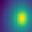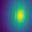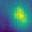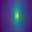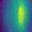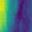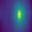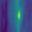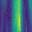Non-pulsar: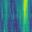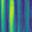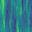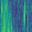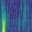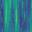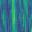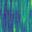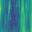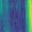## Using the Dataset in PyTorch

The htru1.py file contains an instance of the torchvision Dataset() for the HTRU1 Batched Dataset.

To use it with PyTorch in Python, first import the torchvision datasets and transforms libraries:

``````from torchvision import datasets
import torchvision.transforms as transforms
``````

Then import the HTRU1 class:

``````from htru1 import HTRU1
``````

Define the transform:

``````# convert data to a normalized torch.FloatTensor
transform = transforms.Compose([
transforms.RandomHorizontalFlip(), # randomly flip and rotate
transforms.RandomRotation(10),
transforms.ToTensor(),
transforms.Normalize((0.5, 0.5, 0.5), (0.5, 0.5, 0.5))
])
``````

``````# choose the training and test datasets
``````

### Using Individual Channels in PyTorch

If you want to use only one of the “channels” in the HTRU1 Batched Dataset, you can extract it using the torchvision generic transform transforms.Lambda.

This function extracts a specific channel (“c”) and writes the image of that channel out as a greyscale PIL Image:

``````def select_channel(x,c):

from PIL import Image

np_img = np.array(x, dtype=np.uint8)
ch_img = np_img[:,:,c]
img = Image.fromarray(ch_img, 'L')

return img
``````

`````` transform = transforms.Compose(
[transforms.Lambda(lambda x: select_channel(x,0)),
transforms.ToTensor(),
transforms.Normalize([0.5],[0.5])])
``````

### Jupyter Notebooks

An example of classification using the HTRU1 class in PyTorch is provided as a Jupyter notebook treating the dataset as an RGB image and also extracting an individual channel as a greyscale image.

These are examples for demonstration only - please don’t use them for science!